﻿ Kirchhoff’s LawBack

## Kirchhoff’s Law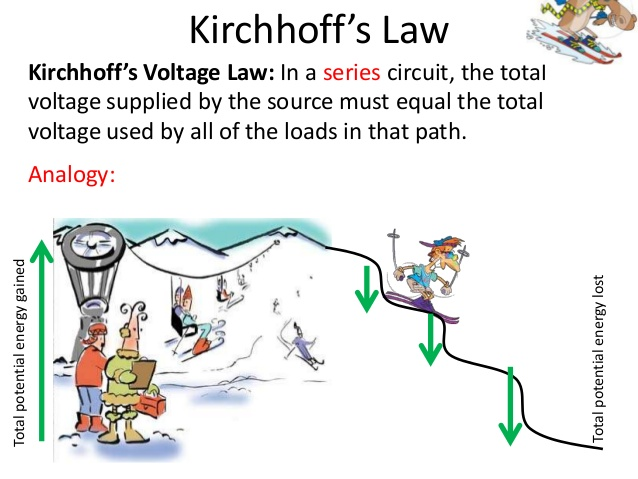## Relationships between U and I

The two Kirchhoff’s Laws tell us about the relationships between voltages and currents in circuits.

"Kirchhoff’s Current Law states that: The algebraic sum of currents at a node is zero."

Two points might need further explanation:

1. A node is the technical term for a junction in a circuit, where two or more branches are joined together. Fig. 2.1 shows a node with four branches connected;
2. the phrase algebraic sum reminds us that we have to take account of the current direction, as well as magnitude, when applying Kirchhoff’s Current Law.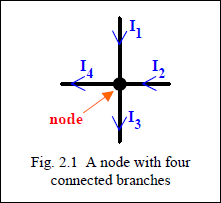This Law is used in circuit analysis to define relationships between currents flowing in branches of the circuit. For example, in Fig. 2.1the currents flowing in the four branches connected to the node have been defined as I1, I2, I3, I4 and Kirchhoff’s Current Law allows us to write down an equation relating these currents.

Looking closely at Fig. 2.1, we see that two of the currents (I1, I2) are flowing towards the node, while the other two currents (I3, I4) are flowing outwards. The ‘algebraic sum’ needs to take account of this difference in relative direction.

To apply Kirchhoff’s Current Law rigorously, we must first make an arbitrary choice of positive current direction.

Suppose currents flowing in to the node (I1, I2) are treated as positive contributions to the algebraic sum (and conversely currents flowing from the node are treated as negative contributions), then the algebraic sum of currents would be written: + I1 + I2 – I3 – I4 , and according to Kirchhoff’s Current Law this algebraic sum is equal to zero:

+ I1 + I2 – I3 – I4 = 0        (2.1)

The same result could be obtained with the opposite choice of positive current direction. If currents flowing from the node (I3, I4) are treated as positive contributions to the algebraic sum, then the algebraic sum of currents would be written: – I1 – I2 + I3 + I4 , and equating this algebraic sum to zero:

– I1 – I2 + I3 + I4 = 0        (2.2)

which is the same relationship as Eqn. 2.1 with all terms multiplied by –1.

It must be emphasised that the choice of sign convention when using Kirchhoff’s Current Law is entirely arbitrary and, of course, makes no difference to the result obtained. However, it is good practice to be consistent in your choice, because this minimises the chance of making a mistake when writing down the algebraic sum.

Eqns. 2.1 and 2.2 can be re-arranged to show that:

I1 + I2 = I3 + I4 (2.3)

and referring back to Fig. 2.1 we see that this equation is showing that current flowing into the node is equal to the current flowing out. This formulation arises naturally from physical considerations of current as the flow of charge.

Charge does not accumulate at a node and therefore any charge flowing into the node through one or more branches must flow out from the node through other branches. Therefore, current flowing into is equal to current flowing out of the node.

### Worked example 2.1

Calculate the current I flowing into the node: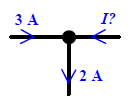#### Solution

Choosing currents flowing into the node as positive and applying Kirchhoff’s

Current Law: +3 –2 +I = 0, so I = -1 A

The current flowing into the node is –1A, which is the same as +1A flowing out of the node

### Worked example 2.2

Calculate the current I defined in the diagram.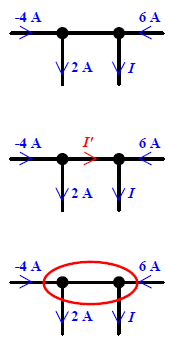#### Solution

In this problem, there are two nodes, each with three branches connected. Begin by defining the current I’ flowing in the branch between the two nodes. The direction of I’ has been chosen randomly: it may turn out to have a positive or negative value. Choosing currents flowing out of the nodes as positive and applying Kirchhoff’s Current Law at each node:

-(-4) + 2 + I’ = 0, so I’ = -6 A

and: -I’ – 6 + I = 0, so I = I’ + 6 = 0 A

but is there any easier way? Yes! We can merge the two separate nodes into a single supernode, shown in red in the lower diagram. The supernode can’t accumulate charge, so Kirchhoff’s Current Law can be applied to currents in branches connected to it.

Making the same choice of current direction:

-(-4) + 2 + I – 6 = 0, so I = 0 A

## Second of Kirchhoff’s Laws

The second of Kirchhoff’s Laws, the Voltage Law, states that:

The algebraic sum of voltages around a closed circuit loop is zero.

There’s the phrase ‘algebraic sum’ again, so we must recognise that the direction of voltages matters when using Kirchhoff’s Voltage Law.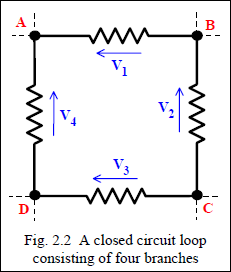Fig. 2.2 shows a circuit loop, which is part of a larger circuit. The loop involves four nodes, ABCD, between which are connected four components. In this case the four components are resistances, but Kirchhoff’s Voltage Law can be applied no matter what components are connected in the closed circuit loop. The voltages across the four resistances comprising the circuit loop have been defined as V1, V2, V3, V4 and Kirchhoff’s Voltage Law allows us to write down an equation relating these voltages. If we think about travelling around the closed circuit loop in any direction, we note that the four voltages will be encountered in sequence.

Two of the voltage arrows will point in the direction of travel and two will oppose the travel. Thealgebraic sum of voltages needs to take account of this difference in relative direction.

To apply Kirchhoff’s Voltage Law correctly, we must make arbitrary choices about the direction of travel around the closed circuit loop and the contribution which the separate voltages make to the algebraic sum around the closed circuit loop. Suppose we travel around the loop in Fig. 2.2 in the clockwise direction (ABCD) and that voltages opposite to the direction of travel make a positive contribution to the algebraic sum. In travelling from A to B the voltage V1 is encountered and it is in a direction which is opposite to the travel. Therefore, V1 is a positive contribution to the algebraic sum.

The same comment is true of V2, which is met when proceeding from B to C. However, travelling from C to D and back to A, the voltages V3 and V4 are encountered and in both cases the voltages are in the same direction as the travel, giving a negative contribution to the algebraic sum. Expressed mathematically, the algebraic sum of voltages around the closed loop ABCD is: + V1 + V2 – V3 – V4 and Kirchhoff’s Voltage Law states that this sum is equal to zero:

+ V1 + V2 – V3 – V4 = 0 (2.4)

The same result is obtained for any choice of travel direction or voltage contribution to the algebraic sum. The other three combinations are:

Clockwise around the loop (ABCD), with the arrow positive:

– V1 – V2 + V3 + V4 = 0 (2.5)

Anticlockwise around the loop (ADCB), against the arrow positive:

– V1 – V2 + V3 + V4 = 0 (2.6)

Anticlockwise around the loop (ADCB), with the arrow positive:

+ V1 + V2 – V3 – V4 = 0 (2.7)

The four equations 2.4 – 2.7 produce exactly the same relationship between the four voltages: all four can be re-arranged to show that:

V1 + V2 = V3 + V4 (2.8)

As with the Current Law, it is a good idea to be consistent in your choice of direction and polarity when applying Kirchhoff’s Voltage Law, so there is less chance of error when writing down the algebraic sum.

### Worked example 2.3

Calculate the voltage V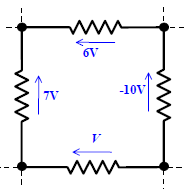#### Solution

With an arbitrary choice of clockwise travel around the loop and counting with the voltage arrow as a positive contribution to algebraic sum, Kirchhoff’s Voltage Law:

-6 – (-10) + V +7 = 0,

so V = -11 V

### Worked example 2.4

Calculate the voltage V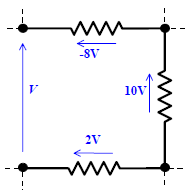#### Solution

This example is intended to demonstrate that the ‘closed circuit loop’ does not need to be defined by a continuous connection of components: the voltage V is the voltage between two nodes, which have nothing connected between them, yet Kirchhoff’s Voltage Law is still valid.

With anticlockwise travel around the loop and counting against the voltage arrow as a positive contribution to algebraic sum:

+ V + 2 – 10 – (-8) = 0, so V = 0 VAnd finally, a brief note on notation. You will find many books referring to the voltage between two points in a circuit, such as A and B, using the symbol VAB.

Naturally, you will wonder how this relates to the ‘arrow’ notation used here. As shown in Fig. 2.3, the convention is that the voltage VAB means ‘the voltage at A relative to B’, so the arrow points at A from B.